# Fix Excel VLOOKUP Not Working [Quick Ways to Resolve 2023]Written By FarhanMax

VLOOKUP is a widespread, popular, and widely used Excel function to quickly find information in a table, rage, or a large spreadsheet.

But, when users do not carefully follow VLOOKUP rules and syntax or of some limitations in the scripts, it gives incorrect results to users and stops working.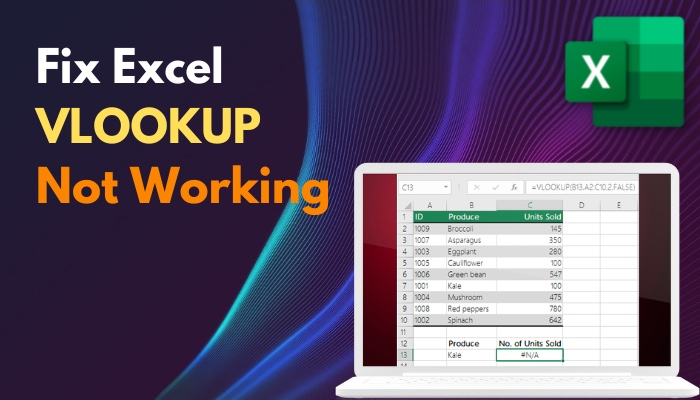In this article, I will discuss some common errors, why VLOOKUP does not work, and how to fix them.

So without further due, let’s jump in.

## How to Fix VLOOKUP Not Working in Microsoft Excel

You need to apply the fixes according to the error codes you see while performing the VLOOKUP in Microsoft Excel. To fix the #N/A error, you need to remove the extra spaces in the Lookup value or solve the #NAME error and check for an incomplete argument in the formula.

However, while performing the fixes, Microsoft Excel can freeze or slow. Follow our guide to solve the issue.

In this part, I will show you the best way to solve the VLOOKUP not working issue in Microsoft Excel. Let’s see how to do it.

Here are the fixes for VLOOKUP Not Working in Microsoft Excel:

### 1. Remove Spaces in Lookup Value

You will get the #N/A error when there is an extra space in your Excel sheet. So when you insert =VLOOKUP(L2,\$I\$1:\$J\$9,2,FALSE), you get a #N/A error in the VLOOKUP.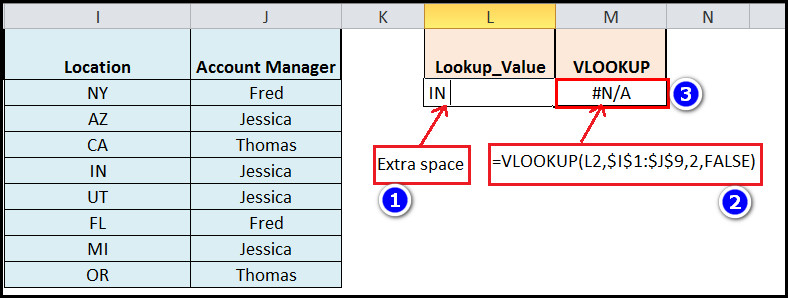To solve this error, follow the steps below:

• Click on the Function box.
• Type or copy paste =VLOOKUP(TRIM(L2),\$I\$1:\$J\$9,2,FALSE).
• Press the Enter key on your keyboard.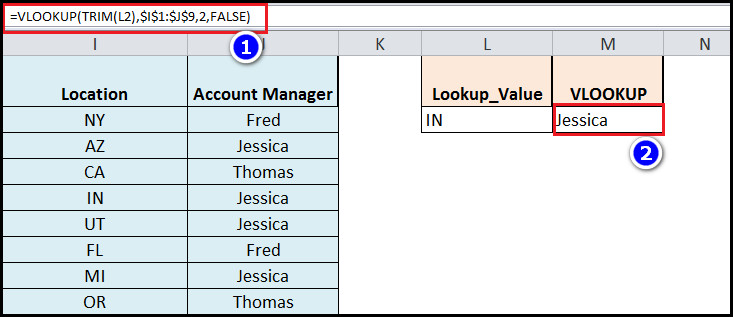You can also copy values without formulas from Microsoft Excel to avoid the issue.

### 2. Type Values in Lookup_Value Argument Currently

When you insert the wrong value in the lookup_value argument of the VLOOKUP function, you will get a #N/A error in the result.

So you should re-check the values to remove the #N/A error in Excel VLOOKUP.

### 3. Change Value Formats

When the Excel file formats numeric values as text in a table_array argument, you will see the #N/A error in the VLOOKUP search results. In that case, you need to format the data properly.

Here are the steps to change the value formats in Excel:

• Open the Excel file and sleet the numeric column.
• Go to the Data ribbon and tap the Texts to columns option.
• Click Next> two times.
• Select the General option in the options window and tap Finish.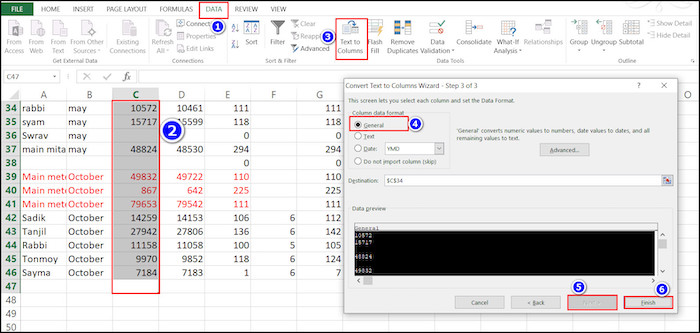After completing the steps, you will see the correct results in VLOOKUP.

### 4. Arrange Lookup Values in the First Column

According to the Microsoft Excel rule, the lookup value needs to be in the first (leftmost) column of a table_array; otherwise, the VLOOKUP feature won’t work.

So you must arrange your columns correctly before selecting your table_array in the VLOOKUP.

### 5. Fix Index_number

If you input the index_number argument less, you will face the #VALUE error in VLOOKUP. That’s why you need to check the index_number argument and make it more than 1.

### 6. Current Workbook Path

If you input the table_array from another workbook while using the VLOOKUP feature, sometimes the path of that workbook becomes incomplete. And for that incomplete workbook path, you will face a #VALUE error in the VLOOKUP.

To solve this issue, type =VLOOKUP(lookup_value, ‘[workbook name]sheet name’!table_array, col_index_num, FALSE) in the functions bar and press the Enter key.

### 6. Meet Characters Length Requirements

The VLOOKUP option of Microsoft Excel only supports 255 characters in the lookup value argument. When you exceed the limit, you will get a #VALUE error.

You can use the INDEX MATCH formula instead of the VLOOKUP to avoid the problem.

### 7. Insert Current Number Of Columns

When you insert an index_number in VLOOKUP greater than the number of columns in the document, you will see a #REF error message in the results.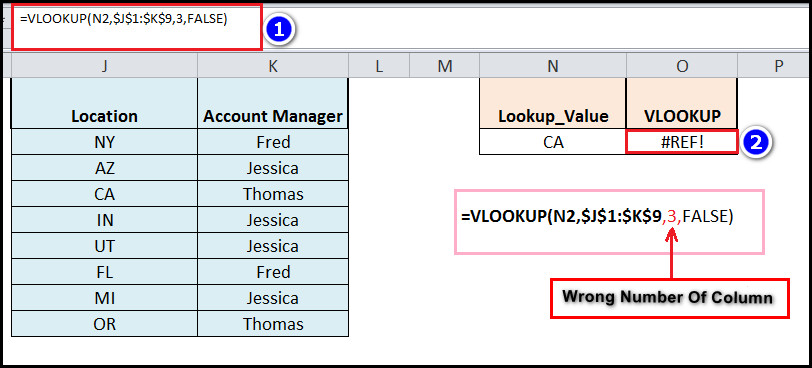So you should check the column number in the function box.

### 8. Use Approximate Match Values

When the VLOOKUP option does not return the correct value, you need to set the line of the function to the approximate value. It will help you find the correct result in your Excel sheet.

Example:
When you don’t get accurate results using =VLOOKUP(L2,\$I\$1:\$J\$9,2,FALSE) option, you need to insert the =VLOOKUP(L2,\$I\$1:\$J\$9,2,TRUE) function key in the box.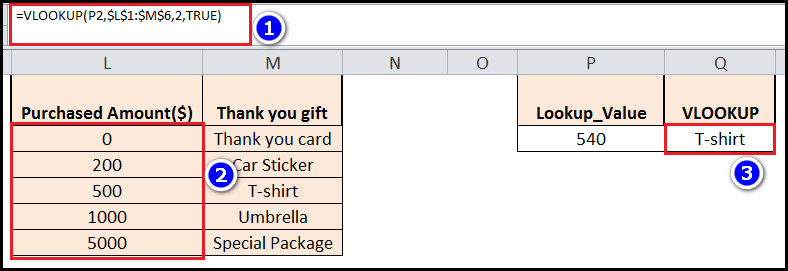After applying the fixes, you won’t face VLOOKUP not working issues in Microsoft Excel again.

## Why is VLOOKUP Stops Working in Microsoft Excel?

Usually, the VLOOKUP error of Microsoft Excel happens when there is some extra space in the data or the Numeric values formats as Text in excel. Also, if you made a typo mistake in Lookup_Value, you will see the #N/A error in excel VLOOKUP.

In this section, I will give you a table indicating the common errors of Vlookup and what causes them in the first palace. It will help you to avoid those mistakes in the first palace.

Here are the common errors of VLOOKUP in Microsoft Excel and what causes them:

Error NameReasons of the Error
#N/A Error● Number stored as text.
● Extra Spaces in Lookup Value.
● Typing mistake in Lookup Value.
● Wrong data range.
● Lookup Value not in the First column.
● Lookup value is smaller than the lowest value.
#VALUE Error ●Index number less than 1.
● Incorrect or incomplete Workbook path.
● Exceeds 255 characters in length.
#REF Error● Index-number argument exceeds the number of columns.
● Missing data.
#NAME Error● Incomplete argument in the formula.
VLOOKUP returning incorrect results● DATA not sorted in ascending order.
● A new column is inserted into the table.

As you can see, the VLOOKUP errors mainly happen for inputting the wrong data or selecting the incorrect range.

If you are careful about those mistakes, then the VLOOKUP errors won’t happen. You can also install Macro in Excel to further enhance the automation process.

## FAQs

Is there an alternative to VLOOKUP?

Yes, you can use INDEX MATCH MATCH instead of VLOOKUP for searching in rows and columns in Microsoft Excel.

Is Xlookup better than VLOOKUP?

If you want an exact data match in an Excel sheet, Xlookup is best. But for an approximate match, VLOOKUP is better.

Which is better, the INDEX match or VLOOKUP?

Index Match is more flexible than VLOOKUP. Because you can search by row, column, or by both with this feature.

## Conclusion

In short, the VLOOKUP usually stops working when you input any incorrect data or unnecessary spaces in Lookup Value. It also happens for script limitations in the functions file or data format errors. You can solve this issue by removing the extra space pr ensuring the correct data format.

After reading the article, I’m sure you now know the methods to solve the VLOOKUP errors in Microsoft Excel. Comment if you face any problems while applying the steps.

And for everything else, stick with 10 Scopes.